# Marsha Foshee

•### Finding the Fourth Vertex of a Parallelogram

Activity

Marsha Foshee

•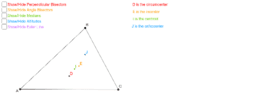### Euler Line and Points of Concurrency

Activity

Marsha Foshee

•### Writing power functions given a table of values

Activity

Marsha Foshee

•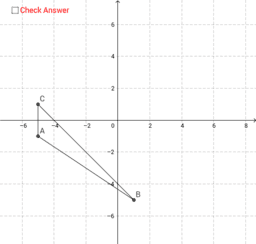### Finding Vertices Given Midpoints

Activity

Marsha Foshee

•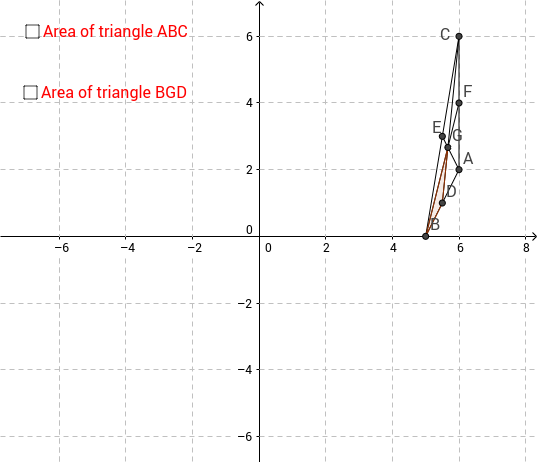### Finding Areas of Triangles using Medians

Activity

Marsha Foshee

•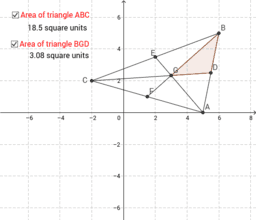### Finding Areas of Triangles using Medians

Activity

Marsha Foshee

•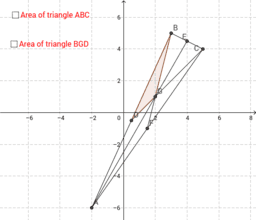### Finding Areas of Triangles using Medians

Activity

Marsha Foshee

•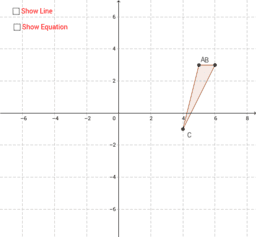### Writing Equations of Median Lines

Activity

Marsha Foshee

•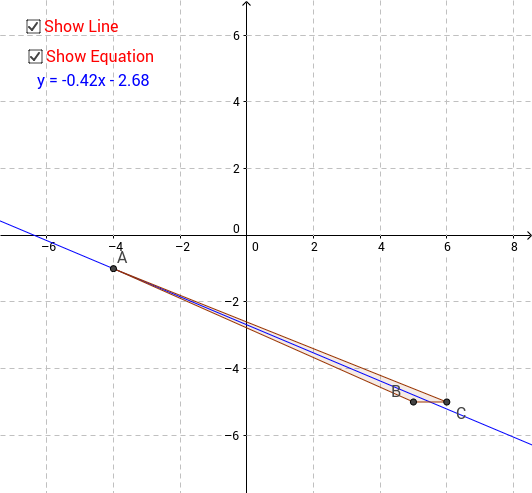### Writing Equations of Median Lines

Activity

Marsha Foshee

•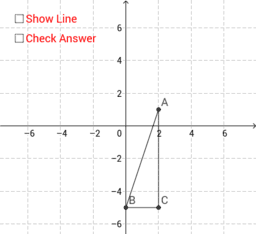### Writing Equations of Altitudes

Activity

Marsha Foshee

•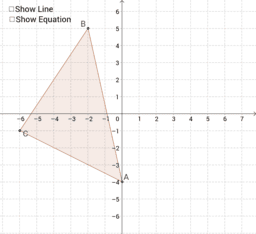### Writing the equation of a median line of a triangle

Activity

Marsha Foshee

•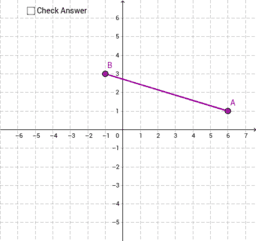### Writing the equation of a perpendicular bisector

Activity

Marsha Foshee

•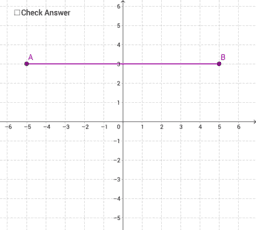### Writing the equation of a perpendicular bisector

Activity

Marsha Foshee